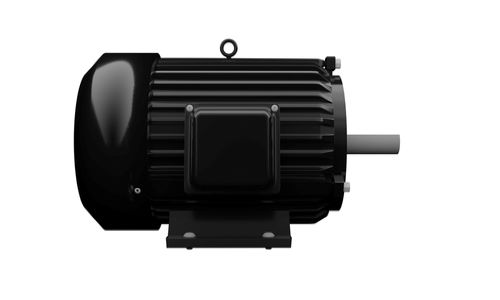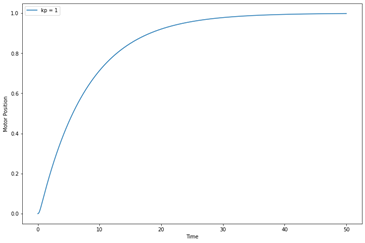# Tuning a DC motor position controllerIn this example, we will show how to design a PID controller for controlling the position of a DC motor shaft. We will apply the Ziegler-Nichols method for empirically tuning the PID controller using Collimator.

## Motor position model

The motor is conceptually modeled as in Figure 1 with the parameters given in Table 1.

Table 1 DC motor model parameters:

\begin{array} {|l|l|}\hline Symbol & Description & Value & Unit \\ \hline R & \text{Shunt Resistance} & 4 & \Omega \\ \hline L & \text{Coil inductance} & .25 & H \\ \hline K & \text{Motor Electromotive/torque constant} & .05 & N.m/A \\ \hline j & \text{Rotor intertia} & .02 & Kg.m2 \\ \hline b & \text{Friction constant}  & 0.1 & N.m.s \\ \hline  \end{array}

The transfer function of the DC motor position to the input voltage is described in the following Equation:

$$\frac{Φ(s)}{U(s)} =\frac{K}{LJs^3+(RJ+Lb) s^2+(Rb+K^2 )s}$$

We can build the simulation diagram for the DC motor position with the PID controller using Collimator's model editor as in Figure 2. We have also added a disturbance to later assess the disturbance rejection capability of the tuned controller.

We configure the DC Motor Position block from the in order to define the transfer function with the following parameters:

$$Numerator= [0.05]$$

$$Denominator= [0.005,0.105,0.4025,0]$$

Then, we add the controller parameters to the model parameters configuration tab:

## PID motor position controller tuning

Before we start analyzing the model and tuning the controller, we will need to import some useful libraries:

import numpy as np
import matplotlib.pyplot as plt
import control as ctrl
import collimator as C
from matplotlib.ticker import AutoMinorLocator



We will also define a function that returns the step response information as overshoot, rising time, and settling time:

def step_info(t,response):
print("Overshoot: %f%s"%((response.max()/response[-1]-1)*100,'%'))
print("Rise time (90%%): %fs"%(t[next(i for i in range(0,len(response)-1) if response[i]>response[-1]*.90)]-t))
print("Settling time (2%%): %fs"%(t[next(len(response)-i for i in range(2,len(response)-1) if abs(response[-i]/response[-1])<0.98)]-t))



The first step is to list the available models within our workspace and define a variable that links the simulation diagram within the notebook.

C.list_models()


[<Model name='DC Motor Position TF'>]


my_model = C.load_model('DC Motor Position TF')


my_model
[<Model name='DC Motor Position TF'>]



Then, we run a test simulation to check that we implemented our model correctly with no syntactical error:

sim = C.run_simulation(my_model)
sim.show_logs()


2022-05-02 15:51:42 INF starting model binary
2022-05-02 15:51:42 INF simulation completed successfully exit code=0 total_time=0.161123 simulation time=0.037949



To tune the PID controller, we begin with a simple P controller with $$K_p=1$$

kp = 1
ki = 0
kd = 0
my_model.set_parameters({"kp":kp, "ki":ki, "kd":kd})
my_model.set_configuration({"stop_time":50})
sim = C.run_simulation(my_model)
my_results = sim.results.to_pandas()
plt.rcParams["figure.figsize"] = (12,8)
plt.plot(my_results['time'],my_results['DCMotorPosition'],label="kp = "+str(kp))
plt.legend()
plt.xlabel("Time")
plt.ylabel("Motor Position")
step_info(my_results['time'].to_numpy(),my_results['DCMotorPosition'].to_numpy())



The step response for the DC motor position with the P controller is shown from the visualizer in Figure 4.Figure 4 Step response for P controller with $$K_p = 1$$

The initially tuned P controller yields a very slow step response:

\begin{array} {|l|l|}\hline \text{Overshoot} & 0.00000\% \\ \hline \text{Raise Time (90%) } & 18.080000s \\ \hline \text{Settling time (2%)} & 30.080000s \\ \hline  \end{array}

Therefore, we will consider the Ziegler-Nichols table for empirical tuning of the PID controller. The Ziegler-Nichols tuning rule is defined in Table 2

Table 2 Ziegler-Nichols tuning rules:

\begin{array} {|l|l|}\hline \text{Controller Type} & Kp & Ki & Kd \\ \hline P & 0.5K_u & 0 & 0 \\ \hline PI & 0.45K_u & 0.54\frac{K_u}{T_u} & 0 \\ \hline PD & 0.8K_u & 0 & 0.1K_uT_u \\ \hline \text{PID} 1 & 0.6K_u & 1.2\frac{K_u}{T_u} & 0.075K_uT_u \\ \hline \text{PID} 2 & 0.33K_u & 0.66\frac{K_u}{T_u} & 0.11K_uT_u \\ \hline \text{PID} 3 & 0.20K_u & 0.40\frac{K_u}{T_u} & 0.066K_uT_u \\ \hline  \end{array}

The tuning rules depend on determining the ultimate gain $$K_u$$ and the oscillation period $$T_u.$$ To determine these values, we perform a sweep for the P gain until we get a pure oscillatory response:

plt.rcParams["figure.figsize"] = (12,8)
plt.axes().xaxis.set_minor_locator(AutoMinorLocator())
plt.grid(which='both')
my_model.set_configuration({"stop_time":5})
for kp in np.array([100, 160, 170, 200]):
my_model.set_parameters({"kp":kp})
sim = C.run_simulation(my_model)
my_results = sim.results.to_pandas()
plt.plot(my_results['time'],my_results['DCMotorPosition'],label="kp = "+str(kp))
plt.legend()
plt.xlabel("Time")
plt.ylabel("Motor Position")



From Figure 5, we can see that the ultimate gain is and the ultimate period is approximately $$T_u. = 0.65$$.

Consequently, we apply the extracted gain to find the PID controller gains as defined in the Ziegler-Nichols tuning rules:

Ku = 170
Tu = 0.65

kp = [0.5*Ku, 0.45*Ku,     0.8*Ku,      0.6*Ku,        0.333*Ku,      0.2*Ku]
ki = [0,      0.54*Ku/Tu,  0,           1.2*Ku/Tu,     0.667*Ku/Tu,   0.4*Ku/Tu]
kd = [0,      0,           0.1*Ku*Tu,   0.075*Ku*Tu,   0.111*Ku*Tu,   0.0667*Ku*Tu]
labels = ["P", "PI", "PD","PID 1", "PID 2", "PID 3"]
plt.rcParams["figure.figsize"] = (12,8)
for i in range(0, len(labels)):
my_model.set_parameters({"kp":kp[i]})
my_model.set_parameters({"ki":ki[i]})
my_model.set_parameters({"kd":kd[i]})
sim = C.run_simulation(my_model)
my_results = sim.results.to_pandas()
plt.plot(my_results['time'],my_results['DCMotorPosition'],label=labels[i])
plt.xlabel("Time")
plt.ylabel("Motor Position")
plt.legend()




From the step responses, we can see that “PID 2” yields the best step response among the available controllers. Therefore, we will have another look at the “PID 2” controller to investigate the step response the characteristics:

i = 4; # "PID 2"
plt.rcParams["figure.figsize"] = (12,8)
plt.grid()
my_model.set_parameters({"kp":kp[i]})
my_model.set_parameters({"ki":ki[i]})
my_model.set_parameters({"kd":kd[i]})
sim = C.run_simulation(my_model)
my_results = sim.results.to_pandas()
plt.plot(my_results['time'],my_results['DCMotorPosition'],label=labels[i])
plt.xlabel("Time")
plt.ylabel("Motor Position")
plt.legend()
step_info(my_results['time'].to_numpy(),my_results['DCMotorPosition'].to_numpy())
my_model.parameters